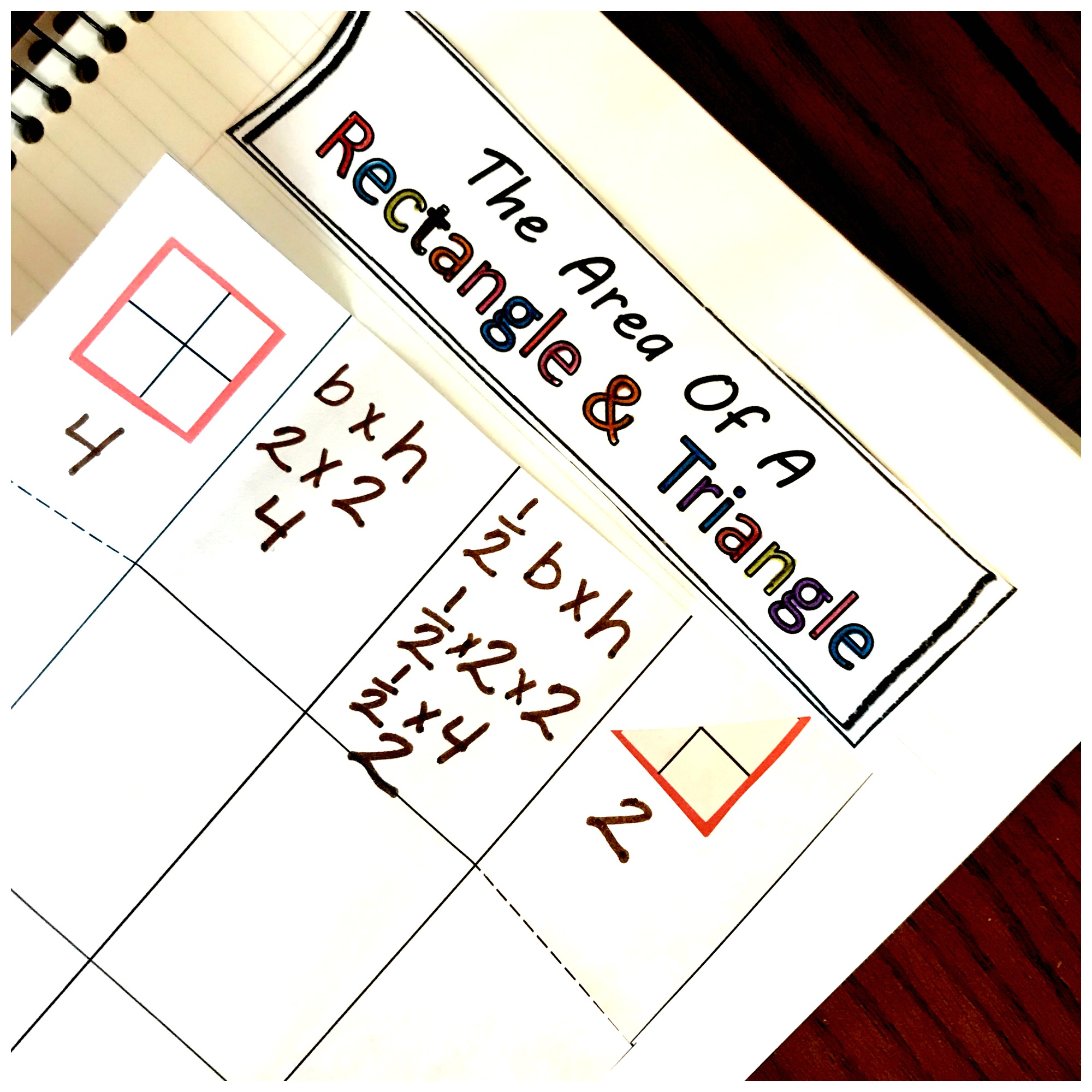Home » Math » A No – Nonsense Interactive Notebook for Area of a Triangle Activitiy

# A No – Nonsense Interactive Notebook for Area of a Triangle Activitiy

This FREE interactive notebook is perfect for helping children understand why the formula for finding the area of a triangle is 1/2 b x h. This is an easy and fun Area of a Triangle Activities.### The free printable can be found at the END of this post. Click on the blue “get your free printable here” button.

We do a lot of singing at home! Little brains can memorize enormous amounts of information, and my boys love to belt out songs as they work through their memory work.

At the beginning of the year, you could hear us skip counting out our songs, but now our focus has changed. We are learning the formulas for area….and I wanted my boys to learn a little about what they were singing.

So it was time for a little Area of Rectangles and Area of Triangle Activities.

You may also like this area and perimeter activity with two different levels:## Prep Work for Interactive Notebook Area Of Triangles Activities

Most of the work for this printable happens during the lesson….so it is easy to get set up.

• First, print off pages.
• Finally, gather up pencils, glue, scissors and math notebooks

## Directions –

#### Set-up1. The first step is to cut out the notebook and glue the middle four squares into the notebook. Cut out the title and glue that at the top of the math notebook page.

2. Next, fold the two flap in. If your kiddos have the time they can decorate the flaps by writing rectangles on the first flap and triangles on the second flap.

3. Finally, cut on the dotted lines

#### Instruction time:The first thing we did was cut out two rectangles or squares that are equal and glued one square in the first box.

Next, we counted up the squares in the shape to figure out the area.

Now it was time to apply for our memory work from cycle 3 week 16 in math. We sang our song about what the area of a rectangle entered and then wrote in our formula.I prefer using b x h, but our memory work has the children learning width times height. They wrote down l x h and I wrote down b x h. This way they could see that both of these formulas work.

Our next step was to use the square to write in the numbers for the formula. It is important to let them know that you are not counting the squares, but the lines (the part you would use to figure out perimeter)

#### Area of a Triangle Time

After figuring out the area of a rectangle, it is time to tackle the triangle. To begin, we cut our square or rectangle in half so that we had two triangles. We glued one in and kept the other triangle nearby.

(When gluing the triangle in, it will work best if you make it a right triangle. It is much easier for the children to see what the height is this way!)

Our next step was to count up the squares in the triangle. Both of the boys struggled with this, so we used the extra triangle. I cut along the grid lines, and then we put the squares together. They were quite surprised how close we came to making complete squares, and this point it was easy to see how many squares the triangle had.

In this example, you can see that the answer is 6.Finally, we hit the last step. The formula for finding the area of a triangle. We sung our song for cycle 3 week 17 math which says that the area of a triangle equals ½ b x h, plugged in our numbers, and solved the problem.

(If your child struggles with figuring out 1/2 of a number, this post may help)

After all that, we discuss why ½ is used when finding the area of a triangle. I hope your children see it as clearly as mine did and they enjoy their area of triangle activities.

You’ve Got This

Rachel